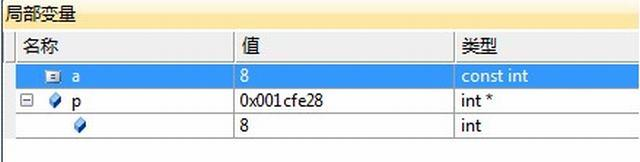# C++ const 关键字小结

const 是 constant 的缩写，本意是不变的，不易改变的意思。在 C++ 中是用来修饰内置类型变量，自定义对象，成员函数，返回值，函数参数。

C++ const 允许指定一个语义约束，编译器会强制实施这个约束，允许程序员告诉编译器某值是保持不变的。如果在编程中确实有某个值保持不变，就应该明确使用const，这样可以获得编译器的帮助。

### 一、const修饰普通类型的变量

```const int  a = 7;
int  b = a; // 正确
a = 8;       // 错误，不能改变```

a 被定义为一个常量，并且可以将 a 赋值给 b，但是不能给 a 再次赋值。对一个常量赋值是违法的事情，因为 a 被编译器认为是一个常量，其值不允许修改。

## 实例

#include
<
iostream
>

using

namespace

std
;
int

main
(
void
)

{

const

int

a
=
7
;
int
*
p
=
(
int
*
)
&
a
; *
p
=
8
;
cout
<<
a
;
system
(

pause

)
;
return

0
;
}Volatile 关键字跟 const 对应相反，是易变的，容易改变的意思。所以不会被编译器优化，编译器也就不会改变对 a 变量的操作。

## 实例

#include
<
iostream
>

using

namespace

std
;
int

main
(
void
)

{

volatile

const

int

a
=
7
;
int
*
p
=
(
int
*
)
&
a
; *
p
=
8
;
cout
<<
a
;
system
(

pause

)
;
return

0
;
}### 二、const 修饰指针变量。

const 修饰指针变量有以下三种情况。

• A: const 修饰指针指向的内容，则内容为不可变量。
• B: const 修饰指针，则指针为不可变量。
• C: const 修饰指针和指针指向的内容，则指针和指针指向的内容都为不可变量。

`const int *p = 8;`

```int a = 8;
int* const p = &a;
*p = 9; // 正确
int  b = 7;
p = &b; // 错误```

```int a = 8;
const int * const  p = &a;```

### 三、const参数传递和函数返回值。

A：值传递的 const 修饰传递，一般这种情况不需要 const 修饰，因为函数会自动产生临时变量复制实参值。

## 实例

#include
<
iostream
>

using

namespace

std
;
void

Cpf
(
const

int

a
)

{

cout
<<
a
;
// ++a; 是错误的，a 不能被改变

}

int

main
(
void
)

{

Cpf
(
8
)
;
system
(

pause

)
;
return

0
;
}

B：当 const 参数为指针时，可以防止指针被意外篡改。

## 实例

#include
<
iostream
>

using

namespace

std
;
void

Cpf
(
int
*
const

a
)

{

cout
<<*
a
<<

; *
a
=
9
;
}

int

main
(
void
)

{

int

a
=
8
;
Cpf
(
&
a
)
;
cout
<<
a
;
// a 为 9

system
(

pause

)
;
return

0
;
}

C：自定义类型的参数传递，需要临时对象复制参数，对于临时对象的构造，需要调用构造函数，比较浪费时间，因此我们采取 const 外加引用传递的方法。

## 实例

#include
<
iostream
>

using

namespace

std
;
class

Test

{

public
:
Test
(
)
{
}

Test
(
int

_m
)
:
_cm
(
_m
)
{
}

int

get_cm
(
)
const

{

return

_cm
;
}

private
:
int

_cm
;
}
;
void

Cmf
(
const

Test
&
_tt
)

{

cout
<<
_tt
.
get_cm
(
)
;
}

int

main
(
void
)

{

Test

t
(
8
)
;
Cmf
(
t
)
;
system
(

pause

)
;
return

0
;
}

Const 修饰返回值分三种情况。

A：const 修饰内置类型的返回值，修饰与不修饰返回值作用一样。

## 实例

#include
<
iostream
>

using

namespace

std
;
const

int

Cmf
(
)

{

return

1
;
}

int

Cpf
(
)

{

return

0
;
}

int

main
(
void
)

{

int

_m
=
Cmf
(
)
;
int

_n
=
Cpf
(
)
;
cout
<<
_m
<<

<<
_n
;
system
(

pause

)
;
return

0
;
}

B: const 修饰自定义类型的作为返回值，此时返回的值不能作为左值使用，既不能被赋值，也不能被修改。

C: const 修饰返回的指针或者引用，是否返回一个指向 const 的指针，取决于我们想让用户干什么。

### 四、const修饰类成员函数

const 修饰类成员函数，其目的是防止成员函数修改被调用对象的值，如果我们不想修改一个调用对象的值，所有的成员函数都应当声明为 const 成员函数。

#include
<
iostream
>

using

namespace

std
;
class

Test

{

public
:
Test
(
)
{
}

Test
(
int

_m
)
:
_cm
(
_m
)
{
}

int

get_cm
(
)
const

{

return

_cm
;
}

private
:
int

_cm
;
}
;
void

Cmf
(
const

Test
&
_tt
)

{

cout
<<
_tt
.
get_cm
(
)
;
}

int

main
(
void
)

{

Test

t
(
8
)
;
Cmf
(
t
)
;
system
(

pause

)
;
return

0
;
}

## 实例

#include
<
iostream
>

using

namespace

std
;
class

Test

{

public
:
Test
(
int

_m
,
int

_t
)
:
_cm
(
_m
)
,
_ct
(
_t
)
{
}

void

Kf
(
)
const

{
++
_cm
;
// 错误

++
_ct
;
// 正确

}

private
:
int

_cm
;
mutable

int

_ct
;
}
;
int

main
(
void
)

{

Test

t
(
8
,
7
)
;
return

0
;
}

www.ysidc.top 西数超哥博客,数据库,西数超哥,虚拟主机,域名注册,域名,云服务器,云主机,云建站,ysidc.top